## Homework Statement

estimate the energy head lost along a short length of pipe suddenly enlarging a diameter of 350mm to 700mm which discharges 0.7(m^-3) of water per second .
the solution given is Q1= Q2 = 0.7(m^3)/s
0.7 = pi ((350x10^-3)^2 ) V1 / 4 , V1= 7.28m/s
0.7 = pi ((700x10^-3)^2 ) V2 / 4 , v2 = 1.82m/s
[(v1)^2 ] / 2g = [(v2)^2 ] / 2g +head loss

## The Attempt at a Solution

the bernoulli's equation is P1/ ρg + (V1^2) /2g + z1 = P2/ ρg + (V2^2) /2g + z2, by writing [(v1)^2 ] / 2g = [(v2)^2 ] / 2g +head loss , the author assume P1 = P2 , how could it be possible ? since the inlet is smaller than outlet , the pressure at inlet should be lower , right ? the velocity at inlet is smaller

Chestermiller
Mentor
The head loss is ##\frac{(P_1-P_2)}{\rho g}##

The head loss is ##\frac{(P_1-P_2)}{\rho g}##
but i dont have P1 and P2.... how to solve this ?

Chestermiller
Mentor
but i dont have P1 and P2.... how to solve this ?
You're solving algebraically for the entire quantity ##\frac{(P_1-P_2)}{\rho g}## (which is the head loss), without having to know either P1 or P2 individually. You are aware that the pipe is assumed to be horizontal so that z1 = z2, correct? And you are aware of the definition of head, correct?

•foo9008
You're solving algebraically for the entire quantity ##\frac{(P_1-P_2)}{\rho g}## (which is the head loss), without having to know either P1 or P2 individually. You are aware that the pipe is assumed to be horizontal so that z1 = z2, correct? And you are aware of the definition of head, correct?
yes , [(v1)^2 ] / 2g = [(v2)^2 ] / 2g +head loss is it correct ??

Chestermiller
Mentor
yes , [(v1)^2 ] / 2g = [(v2)^2 ] / 2g +head loss is it correct ??

the energy flow from point 1 to 2 , so we should add the head loss at point 2 , right ?

Chestermiller
Mentor
The head loss is defined as the decrease in head from the upstream point to the downstream point.

•foo9008
The head loss is defined as the decrease in head from the upstream point to the downstream point.
ya , point 1 is upstream , point 2 is downstream , right ?

The head loss is ##\frac{(P_1-P_2)}{\rho g}##
why the head loss is ##\frac{(P_1-P_2)}{\rho g}##?
shouldn't it be P1/ ρg + (V1^2) /2g + z1 = P2/ ρg + (V2^2) /2g + z2 + head loss ???? we cancel our z1 and z2 , since they are the same

Chestermiller
Mentor
why the head loss is ##\frac{(P_1-P_2)}{\rho g}##?
shouldn't it be P1/ ρg + (V1^2) /2g + z1 = P2/ ρg + (V2^2) /2g + z2 + head loss ???? we cancel our z1 and z2 , since they are the same

•foo9008
A portion of energy is lost to the resistance to flow....

Chestermiller
Mentor
A portion of energy is lost to the resistance to flow....
That is not the general definition of head loss and, in this problem, the resistance to flow is implicitly assumed to be zero. More generally, head loss is the decrease in ##P/(\rho g)+z## between inlet and outlet.

That is not the general definition of head loss and, in this problem, the resistance to flow is implicitly assumed to be zero. More generally, head loss is the decrease in ##P/(\rho g)+z## between inlet and outlet.
can you help how to solve this question ?

That is not the general definition of head loss and, in this problem, the resistance to flow is implicitly assumed to be zero. More generally, head loss is the decrease in ##P/(\rho g)+z## between inlet and outlet.
sorry , i missed out part of thge question . the full question given is
estimate the energy head lost along a short length of pipe suddenly enlarging a diameter of 350mm to 700mm which discharges 0.7(m^-3) of water per second .. If the pressure at entrance is 105N/ (m^2) . Find the pressure at exit

That is not the general definition of head loss and, in this problem, the resistance to flow is implicitly assumed to be zero. More generally, head loss is the decrease in ##P/(\rho g)+z## between inlet and outlet.
so the equation should be (105x10^3)/ (1000x9.81) + (7.28^2) / (2x9.81) +z1 = P2 / (1000x9.81) + (1.82^2) / (2x9.81) + head loss , am i right ?
cancel off z1 and z2 , we still have unknown P2 , how to find the head loss?

Chestermiller
Mentor
so the equation should be (105x10^3)/ (1000x9.81) + (7.28^2) / (2x9.81) +z1 = P2 / (1000x9.81) + (1.82^2) / (2x9.81) + head loss , am i right ?
cancel off z1 and z2 , we still have unknown P2 , how to find the head loss?
No. It's not correct.

$$\frac{P_1}{\rho g}+z_1+\frac{(7.28)^2}{(2)(9.81)}=\frac{P_2}{\rho g}+z_2+\frac{(1.82)^2}{(2)(9.81)}$$
$$\frac{P_1}{\rho g}-\frac{P_2}{\rho g}=head\ loss=\frac{(1.82)^2}{(2)(9.81)}-\frac{(7.28)^2}{(2)(9.81)}=-2.53m$$So,
$$\frac{P_2}{\rho g}=\frac{P_1}{\rho g}+2.53m$$

•foo9008
No. It's not correct.

$$\frac{P_1}{\rho g}+z_1+\frac{(7.28)^2}{(2)(9.81)}=\frac{P_2}{\rho g}+z_2+\frac{(1.82)^2}{(2)(9.81)}$$
$$\frac{P_1}{\rho g}-\frac{P_2}{\rho g}=head\ loss=\frac{(1.82)^2}{(2)(9.81)}-\frac{(7.28)^2}{(2)(9.81)}=-2.53m$$So,
$$\frac{P_2}{\rho g}=\frac{P_1}{\rho g}+2.53m$$
pls refer to the link diagram 6.5 , the formula fo hf is :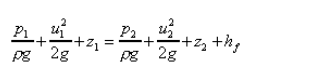http://www.efm.leeds.ac.uk/CIVE/CIVE1400/Examples/eg6_ans.htm

No. It's not correct.

$$\frac{P_1}{\rho g}+z_1+\frac{(7.28)^2}{(2)(9.81)}=\frac{P_2}{\rho g}+z_2+\frac{(1.82)^2}{(2)(9.81)}$$
$$\frac{P_1}{\rho g}-\frac{P_2}{\rho g}=head\ loss=\frac{(1.82)^2}{(2)(9.81)}-\frac{(7.28)^2}{(2)(9.81)}=-2.53m$$So,
$$\frac{P_2}{\rho g}=\frac{P_1}{\rho g}+2.53m$$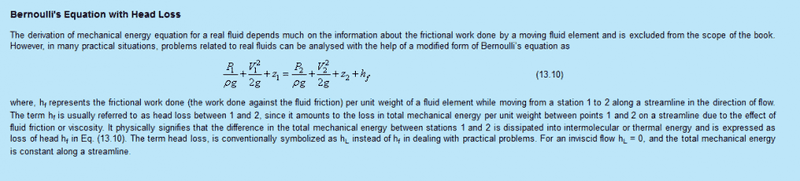No. It's not correct.

$$\frac{P_1}{\rho g}+z_1+\frac{(7.28)^2}{(2)(9.81)}=\frac{P_2}{\rho g}+z_2+\frac{(1.82)^2}{(2)(9.81)}$$
$$\frac{P_1}{\rho g}-\frac{P_2}{\rho g}=head\ loss=\frac{(1.82)^2}{(2)(9.81)}-\frac{(7.28)^2}{(2)(9.81)}=-2.53m$$So,
$$\frac{P_2}{\rho g}=\frac{P_1}{\rho g}+2.53m$$
View attachment 99132

No. It's not correct.

$$\frac{P_1}{\rho g}+z_1+\frac{(7.28)^2}{(2)(9.81)}=\frac{P_2}{\rho g}+z_2+\frac{(1.82)^2}{(2)(9.81)}$$
$$\frac{P_1}{\rho g}-\frac{P_2}{\rho g}=head\ loss=\frac{(1.82)^2}{(2)(9.81)}-\frac{(7.28)^2}{(2)(9.81)}=-2.53m$$So,
$$\frac{P_2}{\rho g}=\frac{P_1}{\rho g}+2.53m$$
is it because when we find the head loss , we pick 2 points which have the same velocity and same pressure.... so we can have only P1 and P2 (2 unknowns) ?
in my calculation , $$\frac{P_1}{\rho g}+z_1+\frac{(7.28)^2}{(2)(9.81)}=\frac{P_2}{\rho g}+z_2+\frac{(1.82)^2}{(2)(9.81)}$$ , i have chose the wrong points , which have different pressure and different velocity , so i am having problems of finding the head loss?

Chestermiller
Mentor
pls refer to the link diagram 6.5 , the formula fo hf is :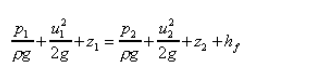http://www.efm.leeds.ac.uk/CIVE/CIVE1400/Examples/eg6_ans.htm
In this equation, hf is the frictional head loss, not the total head loss. The total head loss is h1-h2, where $$h=\frac{p}{\rho g}+z$$

•foo9008
Chestermiller
Mentor
is it because when we find the head loss , we pick 2 points which have the same velocity and same pressure.... so we can have only P1 and P2 (2 unknowns) ?
No. We pick points at the inlet and outlet.
in my calculation , $$\frac{P_1}{\rho g}+z_1+\frac{(7.28)^2}{(2)(9.81)}=\frac{P_2}{\rho g}+z_2+\frac{(1.82)^2}{(2)(9.81)}$$ , i have chose the wrong points , which have different pressure and different velocity , so i am having problems of finding the head loss?
No. This equation is correct. To get the head loss, you don't have to know P1 and P2 individually. You just have to determine their difference. Do you know how to do the algebra to get their difference?

•foo9008
No. We pick points at the inlet and outlet.

No. This equation is correct. To get the head loss, you don't have to know P1 and P2 individually. You just have to determine their difference. Do you know how to do the algebra to get their difference?
as you said in the previous post , The head loss is ##\frac{(P_1-P_2)}{\rho g}## ? so ,
$$\frac{P_1}{\rho g}+z_1+\frac{(7.28)^2}{(2)(9.81)}=\frac{P_2}{\rho g}+z_2+\frac{(1.82)^2}{(2)(9.81)}$$
$$\frac{P_1}{\rho g}-\frac{P_2}{\rho g}=head\ loss=\frac{(1.82)^2}{(2)(9.81)}-\frac{(7.28)^2}{(2)(9.81)}=-2.53m$$So,
$$\frac{P_2}{\rho g}=\frac{P_1}{\rho g}+2.53m$$ ??

Chestermiller
Mentor
as you said in the previous post , The head loss is ##\frac{(P_1-P_2)}{\rho g}## ? so ,
$$\frac{P_1}{\rho g}+z_1+\frac{(7.28)^2}{(2)(9.81)}=\frac{P_2}{\rho g}+z_2+\frac{(1.82)^2}{(2)(9.81)}$$
$$\frac{P_1}{\rho g}-\frac{P_2}{\rho g}=head\ loss=\frac{(1.82)^2}{(2)(9.81)}-\frac{(7.28)^2}{(2)(9.81)}=-2.53m$$So,
$$\frac{P_2}{\rho g}=\frac{P_1}{\rho g}+2.53m$$ ??
Is there a question here?

•foo9008
No. We pick points at the inlet and outlet.

No. This equation is correct. To get the head loss, you don't have to know P1 and P2 individually. You just have to determine their difference. Do you know how to do the algebra to get their difference?
as you said in the previous post , The head loss is ##\frac{(P_1-P_2)}{\rho g}## ? so ,
No. It's not correct.

$$\frac{P_1}{\rho g}+z_1+\frac{(7.28)^2}{(2)(9.81)}=\frac{P_2}{\rho g}+z_2+\frac{(1.82)^2}{(2)(9.81)}$$
$$\frac{P_1}{\rho g}-\frac{P_2}{\rho g}=head\ loss=\frac{(1.82)^2}{(2)(9.81)}-\frac{(7.28)^2}{(2)(9.81)}=-2.53m$$So,
$$\frac{P_2}{\rho g}=\frac{P_1}{\rho g}+2.53m$$
Is there a question here?
that's the correct working for this question , right ? then , i think i understand already , thanks!

Chestermiller
Mentor
as you said in the previous post , The head loss is ##\frac{(P_1-P_2)}{\rho g}## ? so ,

that's the correct working for this question , right ? then , i think i understand already , thanks!
Yes. I said that this is the correct working for the question previously. I think your issue is more with terminology than with the actual application of the methodology.

•foo9008
Yes. I said that this is the correct working for the question previously. I think your issue is more with terminology than with the actual application of the methodology.
https://en.wikipedia.org/wiki/Bernoulli's_principle#Incompressible_flow_equation
head loss is also known as piezometric loss , which has formula of h = P / ρg + z ?????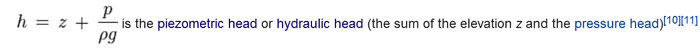but , in my question , it said energy loss , shouldn't it look like this( involve (V^2) /2g ) ?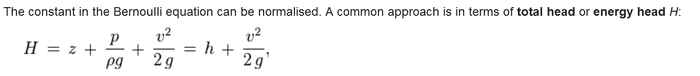Chestermiller
Mentor
https://en.wikipedia.org/wiki/Bernoulli's_principle#Incompressible_flow_equation
head loss is also known as piezometric loss , which has formula of h = P / ρg + z ?????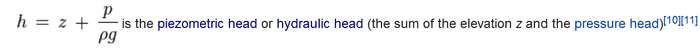but , in my question , it said energy loss , shouldn't it look like this( involve (V^2) /2g ) ?Since you never gave the full exact statement of your problem, I would have to be a clairvoyant to know what it was. Maybe you want to do that now? It might have helped quite a bit if you have given the exact statement from the outset.

•foo9008here it is , it should be 105N/(m^2)

Chestermiller
Mentorhere it is , it should be 105N/(m^2)
Well, it's hard to know what they are referring to by "energy head lost." It isn't clear whether that is the same as "head lost." It also isn't clear whether the "given solution" is correct, because there is actually a head gain calculated , not a head loss.

Also, have you been learning about discharge coefficients and frictional head loss? If so, then the given solution is incorrect. There should be a frictional head loss included in the equation that is calculated from a discharge coefficient, based on the velocity at the 350 mm location (and and the diameter ratio at the sudden enlargement). So, have you been learning about discharge coefficients and, if so, what does your book give for the discharge coefficient for a sudden expansion to twice the diameter?

Well, it's hard to know what they are referring to by "energy head lost." It isn't clear whether that is the same as "head lost." It also isn't clear whether the "given solution" is correct, because there is actually a head gain calculated , not a head loss.

Also, have you been learning about discharge coefficients and frictional head loss? If so, then the given solution is incorrect. There should be a frictional head loss included in the equation that is calculated from a discharge coefficient, based on the velocity at the 350 mm location (and and the diameter ratio at the sudden enlargement). So, have you been learning about discharge coefficients and, if so, what does your book give for the discharge coefficient for a sudden expansion to twice the diameter?
no , i havent learn about discharge coefficient , but , according to the book , the loss due to expansion is given by [(V1 -V2)^2 /] 2g

Well, it's hard to know what they are referring to by "energy head lost." It isn't clear whether that is the same as "head lost." It also isn't clear whether the "given solution" is correct, because there is actually a head gain calculated , not a head loss.

Also, have you been learning about discharge coefficients and frictional head loss? If so, then the given solution is incorrect. There should be a frictional head loss included in the equation that is calculated from a discharge coefficient, based on the velocity at the 350 mm location (and and the diameter ratio at the sudden enlargement). So, have you been learning about discharge coefficients and, if so, what does your book give for the discharge coefficient for a sudden expansion to twice the diameter?
but , if the solution look like the previous post ( your working ) , teh question should be rephrased as estimate the head loss , but not estimate the total head loss/ total energy loss ?

Chestermiller
Mentor
but , if the solution look like the previous post ( your working ) , teh question should be rephrased as estimate the head loss , but not estimate the total head loss/ total energy loss ?
For calculating the frictional head loss at a sudden expansion, see Table 7.5-1 of Transport Phenomena by Bird, Stewart, and Lightfoot.